A Logit Parking Choice Model with Explicit Capacities

Heinz Spiess

EMME/2 Support Center, Haldenstrasse 16, CH-2558 Aegerten, Switzerland

November 1996

Abstract:

In this note we extend the mixed mode logit model for intermediate destination choice to a similar model in which an explicit capacity is associated with each intermediate destination. This leads to a more realistic approach for modeling park&ride trips, since (in contrast to Kiss&Ride trips) the capacity of an intermediate zone is limited by the parking capacity it offers. We first show that this model can be expressed as a convex minimization problem. Then we look at the dual formulation and show that it can be solved efficiently with a coordinate descent method. A solution algorithm is proposed and its properties are discussed in detail. Finally, special attention is given to the practical aspects of applying such a model for real life projects, and a general implementation of the model in the form of a macro for the EMME/2 transportation planning package is outlined.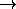First draft! -- Subject to revision!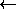Please let me know of any errors and typos you find - thanks!

Introduction

In Spiess (1993) the problem of modeling the intermediate destination choice for mixed mode trips (such as park&ride or kiss&ride) has been discussed as one example of a distribution model based on an activity chain. It was shown how such models, if based on a logit distribution, can be implemented efficiently by the use of simple algebraic matrix multiplications.

The purpose of this note is to focus on the intermediate destination choice for park&ride trips in particular, i.e. on modeling a logit type choice of a convenient parking lot. After a brief recapitulation of the model without parking capacities in section 2, the remainder of this note concentrates on the problem in the presence of explicit capacities at the parking lots and shows how this problem can be formulated and solved efficiently.

While we will refer to the model discussed here specifically as a ``park&ride'' model, it should be noted that the same model is of course also applicable to other kinds of mixed mode travel which impose some capacity constraints at the intermediate destinations. In particular, it can be applied to ferry choice models, such as the one used by the Washington State Ferries (see Dehghani and Gihring, 1995).

Parking choice model without capacities

In the absence of any parking capacity constraints a simple logit type parking lot choice model for park&ride trips is given by the following formula: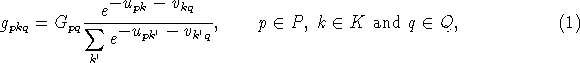where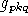is the number of trips between p and q choosing parking site k, according to the (properly weighted) mode specific travel impedances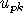for the first leg and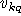for the second leg of the mixed mode trip. The total park&ride demand for O-D pair pq is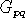.

Note that the above formulation does not include an explicit impedance term for the parking lots (e.g. to model the effects of parking cost). Dropping this term does not cause any loss of generality of the following development, as it can be thought already included in one of the leg impedances.

See Spiess (1993) for a more ``practical'' discussion of this uncapacitated mixed mode model (as a special case of an activity chain model), including details on its implementation using the convolution module of the EMME/2 software (Spiess 1984; INRO 1996). A practical application of this model for the Puget Sound Regional Council has been reported by Blain (1994).

From a mathematical programming point of view, it is important to note that the above parking lot choice model is equivalent to the following convex minimization problem: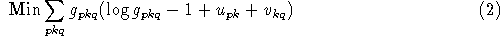subject to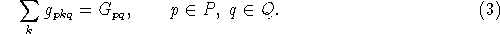By applying the Kuhn-Tucker optimality conditions (see e.g. Luenberger 1984), it can be shown that (1) corresponds indeed to the optimal solution of the above optimization problem.

Parking lot choice model with explicit capacities

We now turn our attention to the parking lot choice model in which an explicit capacity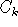is associated with each parking lot k. To formulate the model, we simply add an additional constraint to the uncapacitated problem, yielding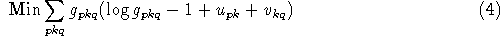subject to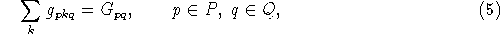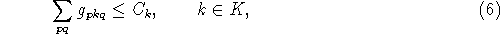In order for the above problem to be feasible, it is of course necessary that the total demand for park&ride trips does not exceed the total amount of parking space available at the parking lots, i.e.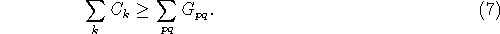The less slack there is in the above inequality, the harder the problem will become to solve. To avoid problems of degeneracy we also assume that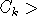0 for all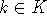.

By introducing the dual variables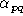for the constraints (5) and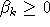for constraints (6) and applying again the Kuhn-Tucker optimality conditions, we obtain a model of the following functional form: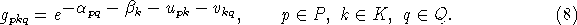subject to (5) and (6).

From this, we can obtain the dual problem formulation as follows: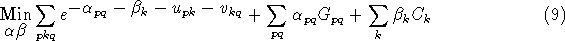subject to,.

The Kuhn-Tucker optimality conditions of the dual problem can be written as follows: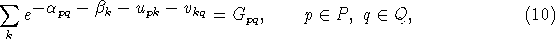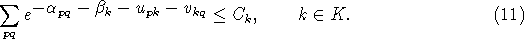The optimal values of the dual variables associated with the parking capacity constraints,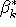, are the shadow prices associated with the ``last'' parking space on parking lot k. They correspond to the additional impedance (or weighted cost) which would need to be imposed at the parking lot in order to defer enough potential trips to other parking lots to meet the given capacity. For parking lots which do not reach capacity (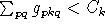) this cost is obviously zero. For a parking lot which is capacity bound,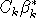can be used as an indicator of the cost imposed to the system by limiting its capacity to.

Solution Algorithm

As the dual problem (9) does not have any explicit constraints, other than the non-negativity of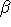, it can be solved efficiently by using the successive coordinate descent method for the dual variables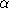and. Thus, the optimal solution to the capacitated parking choice model can, in principle, be found by iteratively solving the equations (10) and (11) forand.

From a practical point of view, it is preferable not to formulate the solution algorithm in terms of the dual variablesand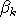, but instead use the following variable substitution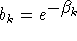and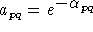. In a similar way, the exponential terms related to the first and second leg impedances can be evaluated ahead of time, which leads to the substitutions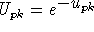and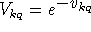. This way, the algorithm will become easier to understand and implement, avoiding the evaluation of any exponentials or logarithms during the execution of the algorithm. In addition, this allows expressing the operations in terms of simple matrix products (as shown in Spiess 1993) -- even though this type of representation is not used here.

Using the above substitutions, a successive coordinate descent algorithm for the dual problem (9) can be formulated as follows:

0.
Set i=1 and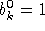for.
1.
Compute parking lot choice based on current values of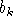: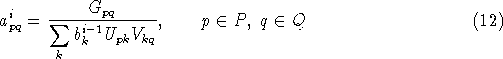and use the results to compute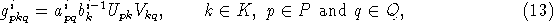2.
Check stopping criterion:
If for allthen STOP.
3.
Compute new values of dual multipliers:4.

From a practical point of view, it is important to note that step 1 of the algorithm corresponds exactly to solving an uncapacitated parking choice model with a parking lot impedance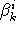. Thus, this step can be implemented using the same procedures or macros which are already available for the problem without capacities.

Even if the above algorithm is terminated before complete convergence is reached, the solution obtained will always satisfy the conservation of flow constraint (5), whereas some of the capacity constraints (6) may still be violated by a certain amount. This is typical for dual based algorithms, and also has the additional advantage that the algorithm will even converge to a solution if the primal problem is infeasible, i.e. if the total park&ride demand exceeds the available total parking space (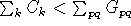). In the later case, the algorithm simply converges to a solution in which --loosely speaking-- the parking capacity constraints are violated the least possible.

If they are needed, the shadow prices can be computed as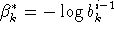once the algorithm has terminated. These values (converted back into cost or impedance values by dividing by the appropriate model coefficients) can be very useful for analyzing the sensitivity of existing capacities or for deciding where to locate additional parking capacity.

Practical Model Implementation

While the previous sections looked at the posed problem from an abstract and theoretical point of view, let us now turn our attention to the practical implementation and use of such a model.

First, it is important to note that the variablesused in the mathematical formulation are not easy to handle in real life (there are far too many of them!), nor are they really pertinent in practice. The problem, as it is posed in practice, is to split the given park&ride demand matrixinto two intermediate demand matrices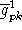and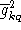:for the first leg (usually the auto part) of the trip, andfor the second leg (usually the transit part). These intermediate matrices can be obtained as follows by using the before-mentioned substitutions in (8) and summing over p or q: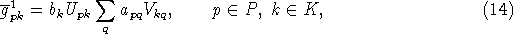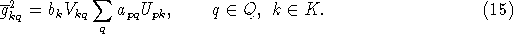Fortunately, the explicit knowledge of the variableis also not required for carrying out the solution algorithm, it suffices to know the values for the total parking lot usage for each lot, which can easily be obtained from either first or second leg demand matrix as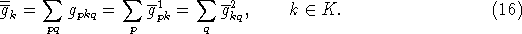The model and the solution algorithm described in the previous sections have been implemented in the EMME/2 transportation planning package (Spiess 1984, INRO 1996) in the form of a macro entitled PARKRIDE. This macro uses the matrix convolution module of EMME/2 to implement the algebraic matrix multiplications (i.e. the sums) in (12), (14) and (15) and the matrix calculator module to compute all other (element by element) operations. For improved efficiency, only the first leg matrixis computed at each iteration of the algorithm. The second leg matrixneeds only be computed once, at the very end of the algorithm. Furthermore, the macro allows the explicit specification of fixed impedance or disutility term for each parking lot, which e.g. can be used for representing parking costs.

The macro PARKRIDE is available via Internet on the Web site of the EMME/2 Support Center.

Conclusions

We have shown that the problem of a logit type intermediate destination choice for mixed mode trips can indeed be extended to include capacity constraints. The resulting model is ``well behaved'' in all important aspects: It has a consistent mathematical formulation, a solution algorithm which is known to produce an optimal solution and which can be (and has been) implemented efficiently even for large scale real-life applications.

References

1
Blain L. (1994). Park-and-Ride Choice using Matrix Convolutions. Paper presented at the 9th Annual International EMME/2 Users Group Conference, Montreal, Quebec.

2
Dehghani Y. and Gihring C. (1995) An Overview of the Washington State Ferries EMME/2 Modeling System. Paper presented at the 10th Annual International EMME/2 Users Group Conference, Portland, Oregon. (TRB/TRR paper forthcoming)

3
INRO Consultants Inc. (1996), EMME/2 User's Manual.

4
Luenberger D.G. Linear and Nonlinear Programming. Second Edition, Addison-Wesley, 1984.

5
Spiess H. (1984). Contributions à la théorie et aux outils de planification de réseaux de transport urbain. Ph.D. thesis, Département d'informatique et de recherche opérationnelle, Centre de recherche sur les transports, Université de Montréal, Publication 382.

6
Spiess H. (1993). Computing Activity Chain Based Trip Distribution Models. EMME/2 Support Center, CH-2558 Aegerten.
...
EMME/2 Support Center, Haldenstrasse 16, CH-2558 Aegerten, Switzerland

Heinz Spiess, EMME/2 Support Center, Tue Nov 12 12:02:04 MET 1996